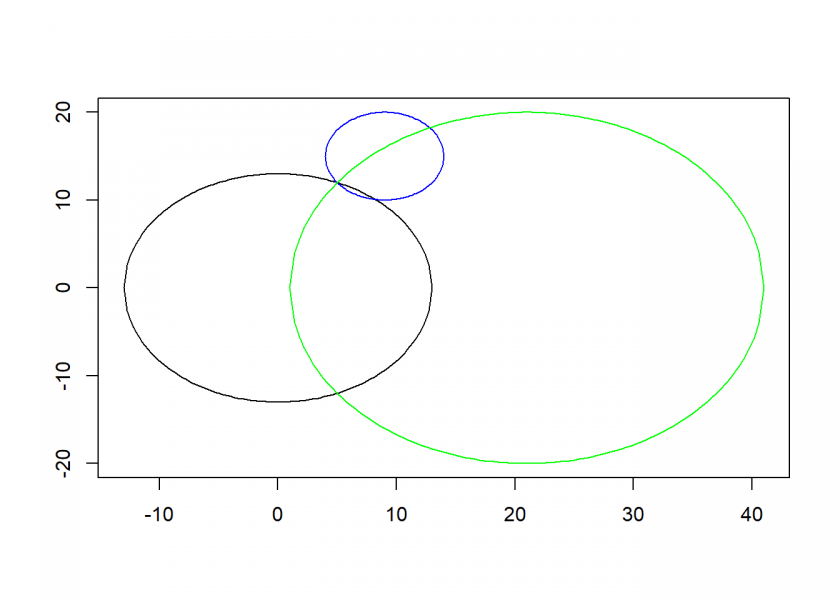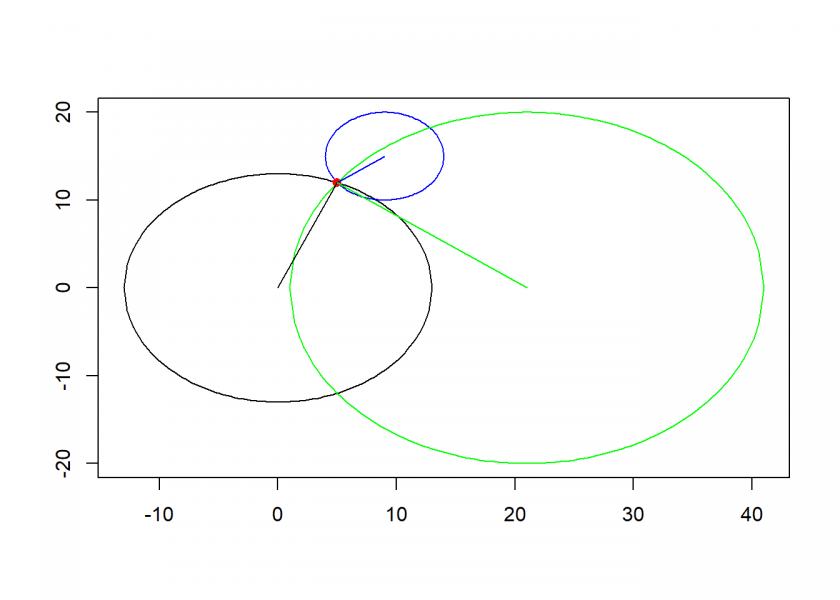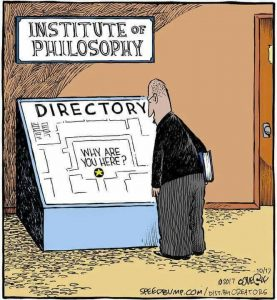Want to share your content on R-bloggers? click here if you have a blog, or here if you don't.Last week, I showed you a method of how to find the fastest path from A to B: Finding the shortest Path with Dijkstra’s Agorithm. To make use of that, we need a method to determine our position at any point in time.

For that matter, many devices use the so-called Global Positioning System (GPS). If you want to understand how it works and do some simple calculations in R, read on!

Nowadays most of us have several GPS devices, in their cars, their smartphones, and their smartwatches. Those are receivers of signals coming from over 30 satellites orbiting the earth. You need that many so that there are always enough in sight:

Many of the technical details are interesting, yet here I want you to understand the core principle of how it works. With the real GPS, you need three satellites to determine two coordinates one of which can be discarded. Because the clock in the GPS receiver is not fully synchronized you need a fourth satellite to compensate for that. The resulting system of equations has to be solved to determine the position. In our toy example, we will only do the 2D-case with two satellites, yet the principle stays the same.

The following example is taken from the excellent article From Barns to Satellites: An Introduction to the Mathematics of Global Positioning Systems by my colleague Professor Kyle Schultz from the University of Mary Washington.

The basic principle of determining the position is finding the intersection of the two circles of the two satellites (in this case) and matching this with a third circle, given by the radius of the earth. We get circles because when we receive the signal of a satellite we receive the time when it sent the signal and the position where it was when it sent the signal. From that, we can infer a circle of all the positions the signal could have traveled to during the time interval until we received it.

When we have two satellites we get two circles. Two circles have either no, one or two intersections. When the system is set up correctly we will have two intersections most of the time. One of those can in nearly all cases be discarded because it will be somewhere in space or beneath the earth (x and y are the coordinates, r is the radius of the circle):

draw_circle <- function(x = 0, y = 0, r, xlim = NULL, ylim = NULL, col = "black", add = FALSE) {
h <- x
k <- y
if (is.null(ylim)) ylim <- c(k - r, k + r)
curve(+ sqrt(r^2 - (x - h)^2) + k, h - r, h + r, xlim = xlim, ylim = ylim, xlab = "", ylab = "", col = col, add = add)
curve(- sqrt(r^2 - (x - h)^2) + k, h - r, h + r, xlim = xlim, ylim = ylim, xlab = "", ylab = "", col = col, add = TRUE)
}

earth <- c(x =  0, y =  0, r = 13) # earth
sat_1 <- c(x = 21, y =  0, r = 20) # satellite 1
sat_2 <- c(x =  9, y = 15, r =  5) # satellite 2

draw_circle(r = earth["r"], xlim = c(-13, 41), ylim = c(-20, 20)) # earth
draw_circle(x = sat_1["x"], y = sat_1["y"], r = sat_1["r"], xlim = c(-13, 41), ylim = c(-20, 20), col = "green", add = TRUE) # satellite 1
draw_circle(x = sat_2["x"], y = sat_2["y"], r = sat_2["r"], ylim = c(-20, 20), col = "blue", add = TRUE) # satellite 2The method for finding the intersection is called trilateration. You can find the exact mathematical derivation in the above article. We put the resulting formula into an R function (the d‘s stand for distances, the p, q and r for the coordinates of the satellites (we do not need the y-value of the first satellite because of the alignment of the diagram, see the article for details!):

trilaterate <- function(d1, d2, d3, p, q, r) {
x <- (p^2 + d1^2 - d2^2) / (2 * p)
y <- (q^2 + r^2 + d1^2 - d3^2 - (q * (p^2 + d1^2 - d2^2)) / p) / (2 * r)
return(c(x, y))
}

(P <- trilaterate(earth["r"], sat_1["r"], sat_2["r"], sat_1["x"], sat_2["x"], sat_2["y"])) # don't need sat_1["y"] because of alignment of satellites with earth
##  x  y
##  5 12

lines(c(earth["x"], P["x"]), c(earth["y"], P["y"]))
lines(c(sat_1["x"], P["x"]), c(sat_1["y"], P["y"]), col = "green")
lines(c(sat_2["x"], P["x"]), c(sat_2["y"], P["y"]), col = "blue")
points(P["x"], P["y"], pch = 16, col = "red")As you can see, we got a unique position (5, 12) by this method.Another fascinating aspect of GPS is, that it is a practical application of Einstein’s Theory of Relativity! That is because the satellites are moving very fast in relation to the surface of the earth and gravity is much weaker up there! Both have to be incorporated into the equation, otherwise, we would have an accumulating error of more than 10 km per day! The system would obviously be totally useless without Einstein and his brilliant theory!